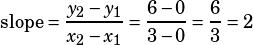Test 7 Section 4 Number 28

I love this question. See how the given line is going through the origin? That means that all the other points on that line will have the same ratio of t to s!

Remember that any two points on a line tell you its slope. When you know a line goes through the origin, then you know one of those points is (0, 0). So using the other point this question gives us, (3, 6), we can calculate the slope:That will hold for all other points on the line!* If the point (s, t) is on this line, then the ratio of t to s will be 2 to 1.

* …other than the origin because of the impossibility of dividing by zero…